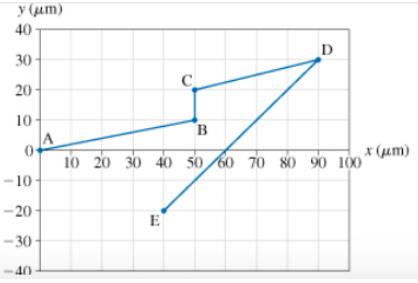# Problem: The bacterium Escherichia coli (or E. coli) is a single-celled organism that lives in the gut of healthy humans and animals. When grown in a uniform medium rich in salts and amino aids, these bacteria swim along zig-zag paths at a constant speed of 20 μm/s. The figure shows the trajectory of an E. coli as it moves from point A to point E. Each segment of the motion can be identified by two letters, such as segment BC.Part A. For the segment AB in the bacterium’s trajectory, calculate the y component of its velocity.Part B. For the segment BC in the bacterium’s trajectory, calculate the x component of its velocity

###### FREE Expert Solution

The graph shown is a position graph. It does not include the time. The velocity vector is in the same direction as the displacement vector shown in the figure.

2D vector Direction:

$\overline{){\mathbf{tan}}{\mathbit{\theta }}{\mathbf{=}}\frac{{\mathbit{A}}_{\mathbit{y}}}{{\mathbit{A}}_{\mathbit{x}}}}$

2D vector component:

89% (209 ratings)###### Problem Details

The bacterium Escherichia coli (or E. coli) is a single-celled organism that lives in the gut of healthy humans and animals. When grown in a uniform medium rich in salts and amino aids, these bacteria swim along zig-zag paths at a constant speed of 20 μm/s. The figure shows the trajectory of an E. coli as it moves from point A to point E. Each segment of the motion can be identified by two letters, such as segment BC.Part A. For the segment AB in the bacterium’s trajectory, calculate the y component of its velocity.

Part B. For the segment BC in the bacterium’s trajectory, calculate the x component of its velocity